# Titanic Kaggle Challenge

My contribution towards the Titanic Challenge on Kaggle.

The goal is to predict the survival rate of passengers given data sets about the information about the passengers onboard.

I used the method of K-Nearest Neighbours to predict the survival rate of the passengers.

References (Taken help from):

The original tutorial submission of titanic data set used the concept of Random Forest Classifier to predict the survived passengers. Base score we get is: 0.77511

I experimented on the same notebook using various other classification models such as Extra Trees Classifiers, ADABoost Classifier and Logistic Regression. However none of the models gave me a better score than the Random Forest Classifier. This meant that the solution to increasing the score was not using different models, but applying some modifications and feature selection to the existing data set.

Original Score: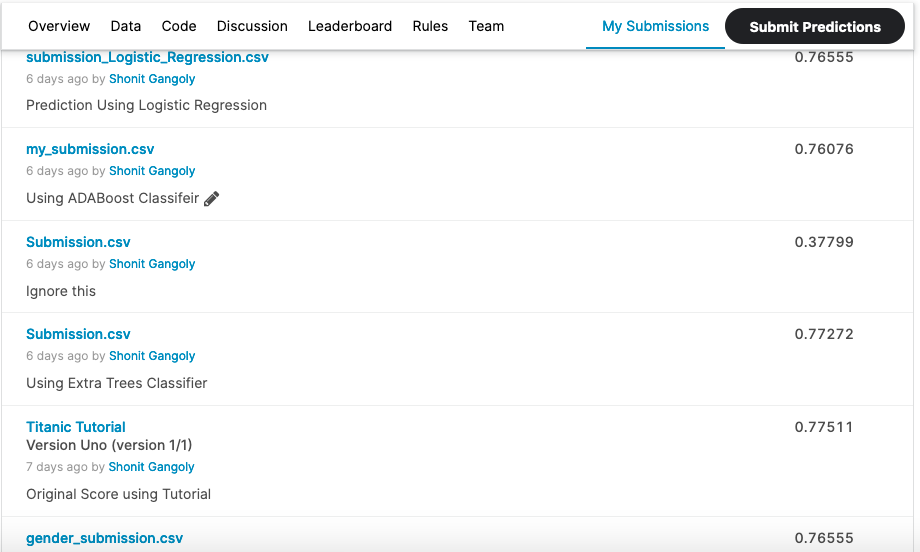Scores using the above classifiers

For my final contribution:

• I used the concepts of pandas qcut, Label Fit Transformers and used KNN Classifier to train the model.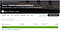Final Score

I started by importing various required modules to work on the data set

`import numpy as npimport pandas as pdimport seaborn as snsfrom matplotlib import pyplot as pltfrom sklearn.preprocessing import StandardScalerfrom sklearn.model_selection import GridSearchCVfrom sklearn.preprocessing import LabelEncoderfrom sklearn.ensemble import RandomForestClassifierfrom sklearn.neighbors import KNeighborsClassifierfrom sklearn import feature_selectionfrom sklearn import model_selectionfrom sklearn import metricsfrom IPython.display import Imagefrom IPython.core.display import HTML`

Next step is to read all the .csv files provided and storing it onto a data frame

`train_df = pd.read_csv("./train.csv")test_df = pd.read_csv("./test.csv")data = train_df.append(test_df)`

Now reading the data files and getting the output

`train_df.head()test_df.head()`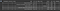Training Data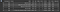Test Data

Now heading towards doing some Exploratory Data Analysis. This is to visualize the data we have and know what steps to take in order to manipulate the data to fit it onto a good model and gain a good accuracy score.

`women = train_df.loc[train_df.Sex == 'female']["Survived"]rate_women = sum(women)/len(women)men = train_df.loc[train_df.Sex == 'male']["Survived"]rate_men = sum(men)/len(men)print("% of men who survived:", rate_men)print("% of women who survived:", rate_women)`Men Survival RateWomen Survival Rate

Next visualize it using some bar graphs. For that, a function stating the required columns is needed and is used to plot according to various other factors

`def bar_chart(feature):survived = train_df[train_df['Survived']==1][feature].value_counts()dead = train_df[train_df['Survived']==0][feature].value_counts()df = pd.DataFrame([survived,dead])df.index = ['Survived','Dead']df.plot(kind='bar',stacked=True, figsize=(15,7))bar_chart('Sex')`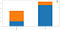Survival according to Sex
`bar_chart('Pclass')bar_chart('SibSp')bar_chart('Parch')bar_chart('Embarked')`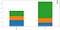Survival according to Class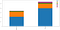Survival according to Siblings and Spouses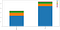Survival according to Children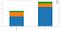Survival according to Passengers who embarked

Now we use the given data and try and add some new columns such that it will help us fit onto our KNN Model better

First Checking the titles the passengers hold

`data['Title'] = data['Name']for name_string in data['Name']:data['Title'] = data['Name'].str.extract('([A-Za-z]+)\.', expand=True)data['Title'].value_counts()`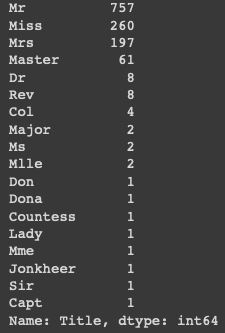Titles

1) Adding all the titles to a string array. This will help in grouping the passenger by their titles. Title carries significance because it gives us the Richness Status of the passenger. The richer the passenger, more the chances of that passenger surviving

2) Adding median values to all the missing entries in the age column

`title_changes = {'Mlle': 'Miss', 'Major': 'Mr', 'Col': 'Mr', 'Sir': 'Mr', 'Don': 'Mr', 'Mme': 'Miss','Jonkheer': 'Mr', 'Lady': 'Mrs', 'Capt': 'Mr', 'Countess': 'Mrs', 'Ms': 'Miss', 'Dona': 'Mrs'}data.replace({'Title': title_changes}, inplace=True)titles = ['Dr', 'Master', 'Miss', 'Mr', 'Mrs', 'Rev']for title in titles:age_to_impute = data.groupby('Title')['Age'].median()[titles.index(title)]data.loc[(data['Age'].isnull()) & (data['Title'] == title), 'Age'] = age_to_imputetrain_df['Age'] = data['Age'][:891]test_df['Age'] = data['Age'][891:]data.drop('Title', axis = 1, inplace = True)`

Creating array called Family Size containing Children, Sibling and Spouse data columns

`data['Family_Size'] = data['Parch'] + data['SibSp']train_df['Family_Size'] = data['Family_Size'][:891]test_df['Family_Size'] = data['Family_Size'][891:]`

1) Reordering passenger names

2) Adding mean values to missing fare values

3) Creating a Family Survival Value that indicates the family survival rate. Setting default as 0.5

`data['Last_Name'] = data['Name'].apply(lambda x: str.split(x, ","))data['Fare'].fillna(data['Fare'].mean(), inplace=True)DEFAULT_SURVIVAL_VALUE = 0.5data['Family_Survival'] = DEFAULT_SURVIVAL_VALUE`

Locating all passengers with Family survival information available. This will help in inducing whether the passenger is alone or not or has family.

`for grp, grp_df in data[['Survived','Name', 'Last_Name', 'Fare', 'Ticket', 'PassengerId','SibSp', 'Parch', 'Age', 'Cabin']].groupby(['Last_Name', 'Fare']):if (len(grp_df) != 1):for ind, row in grp_df.iterrows():smax = grp_df.drop(ind)['Survived'].max()smin = grp_df.drop(ind)['Survived'].min()passID = row['PassengerId']if (smax == 1.0):data.loc[data['PassengerId'] == passID, 'Family_Survival'] = 1elif (smin==0.0):data.loc[data['PassengerId'] == passID, 'Family_Survival'] = 0print("Number of passengers with family survival information:",data.loc[data['Family_Survival']!=0.5].shape)`

Finding passenger who either have families or are in groups by sorting by ticket number

`for _, grp_df in data.groupby('Ticket'):if (len(grp_df) != 1):for ind, row in grp_df.iterrows():if (row['Family_Survival'] == 0) | (row['Family_Survival']== 0.5):smax = grp_df.drop(ind)['Survived'].max()smin = grp_df.drop(ind)['Survived'].min()passID = row['PassengerId']if (smax == 1.0):data.loc[data['PassengerId'] == passID, 'Family_Survival'] = 1elif (smin==0.0):data.loc[data['PassengerId'] == passID, 'Family_Survival'] = 0print("Number of passenger with family/group survival information: "+str(data[data['Family_Survival']!=0.5].shape))train_df['Family_Survival'] = data['Family_Survival'][:891]test_df['Family_Survival'] = data['Family_Survival'][891:]`

1) Using qcut to arrange Fare values. This helps in distributing data according to quartiles.

2) Using Label Fit Transform, this helps in normalizing data

`data['Fare'].fillna(data['Fare'].median(), inplace = True)data['FareBin'] = pd.qcut(data['Fare'], 5)label = LabelEncoder()data['FareBin_Code'] = label.fit_transform(data['FareBin'])train_df['FareBin_Code'] = data['FareBin_Code'][:891]test_df['FareBin_Code'] = data['FareBin_Code'][891:]train_df.drop(['Fare'], 1, inplace=True)test_df.drop(['Fare'], 1, inplace=True)data['AgeBin'] = pd.qcut(data['Age'], 4)label = LabelEncoder()data['AgeBin_Code'] = label.fit_transform(data['AgeBin'])train_df['AgeBin_Code'] = data['AgeBin_Code'][:891]test_df['AgeBin_Code'] = data['AgeBin_Code'][891:]train_df.drop(['Age'], 1, inplace=True)test_df.drop(['Age'], 1, inplace=True)`

1) Normalizing ‘Sex’ data to replace string values with integers. 0 for male, 1 for female

2) Dropping non-normalised columns

`train_df['Sex'].replace(['male','female'],[0,1],inplace=True)test_df['Sex'].replace(['male','female'],[0,1],inplace=True)train_df.drop(['Name', 'PassengerId', 'SibSp', 'Parch', 'Ticket', 'Cabin','Embarked'], axis = 1, inplace = True)test_df.drop(['Name','PassengerId', 'SibSp', 'Parch', 'Ticket', 'Cabin','Embarked'], axis = 1, inplace = True)`

Now checking our newly made columns

Now we split data into two halves. One for training model and the other for testing the model

We also apply standardization to the data columns as standardization of a dataset is a common requirement for many machine learning estimators: they might behave badly if the individual features do not more or less look like standard normally distributed data

`X = train_df.drop('Survived', 1)y = train_df['Survived']X_test = test_df.copy()std_scaler = StandardScaler()X = std_scaler.fit_transform(X)X_test = std_scaler.transform(X_test)`

Now we have to apply our KNN model. To do so, we need to setup required parameters for the model

`n_neighbors = [6,7,8,9,10,11,12,14,16,18,20,22]algorithm = ['auto']weights = ['uniform', 'distance']leaf_size = list(range(1,50,5))hyperparams = {'algorithm': algorithm, 'weights': weights, 'leaf_size': leaf_size,'n_neighbors': n_neighbors}gd=GridSearchCV(estimator = KNeighborsClassifier(), param_grid = hyperparams, verbose=True,cv=10, scoring = "roc_auc")gd.fit(X, y)print(gd.best_score_)print(gd.best_estimator_)`

Here,

1) ‘n_neighbors‘ are the number of neighbors that will vote for the class of the target point

2) For the ‘uniform‘ weight, each of the k neighbors has equal vote whatever its distance from the target point. If the weight is ‘distance‘ then voting weightage or importance varies by inverse of distance; those points who are nearest to the target point have greater influence than those who are farther away.

`gd.best_estimator_.fit(X, y)y_pred = gd.best_estimator_.predict(X_test)knn = KNeighborsClassifier(algorithm='auto', leaf_size=26, metric='minkowski',metric_params=None, n_jobs=1, n_neighbors=6, p=2,weights='uniform')knn.fit(X, y)y_pred = knn.predict(X_test)`

The parameters are as follows:

1) Weights- Uniform weights. All points in each neighborhood are weighted equally.

2) Algorithm- Auto will attempt to decide the most appropriate algorithm based on the values passed to fit method.

3) Leaf Size- Affects the speed of the construction and query, as well as the memory required to store the tree.

4) Power- When p = 2, this is equivalent to using Euclidean_distance

5) Using Minkowski Tree

6) njobs- The number of parallel jobs to run for neighbors search

Now the only thing left is to create an output from our model and generate a .csv file that can be submitted to Kaggle

`temp = pd.DataFrame(pd.read_csv("./test.csv")['PassengerId'])temp['Survived'] = y_predtemp.to_csv("KNN_submission.csv", index = False)`

After submitting it, we can see a score of 0.81818 on Kaggle which is an improvement over the 0.77511 score after following the tutorial methodKaggle Submission

Software Engineer in the making

## More from Shonit Gangoly

Software Engineer in the making